## Selina Concise Mathematics class 7 ICSE Solutions – Simple Interest

APlusTopper.com provides step by step solutions for Selina Concise ICSE Solutions for Class 7 Mathematics. You can download the Selina Concise Mathematics ICSE Solutions for Class 7 with Free PDF download option. Selina Publishers Concise Mathematics for Class 7 ICSE Solutions all questions are solved and explained by expert mathematic teachers as per ICSE board guidelines.

POINTS TO REMEMBERQuestion 1.
Find the S.I. and amount on :
(i) Rs. 150 for 4 years at 5% per year.
(ii) Rs. 350 for 3$$\frac {1 }{ 2 }$$ years at 8% p.a.
(iii) Rs. 620 for 4 months at 8 p. per rupee per month.
(iv) Rs. 3,380 for 30 months at 4 $$\frac { 1 }{ 2 }$$ % p.a.
(v) 600 from July 12 to Dec. 5 at 10% p.a.
(vi) Rs. 850 from 10th March to 3rd August at 2 $$\frac { 1 }{ 2 }$$ % p.a.
(vii) Rs. 225 for 3 years 9 months at 16% p.a.

Solution: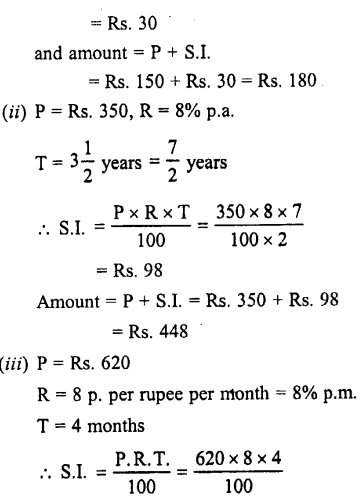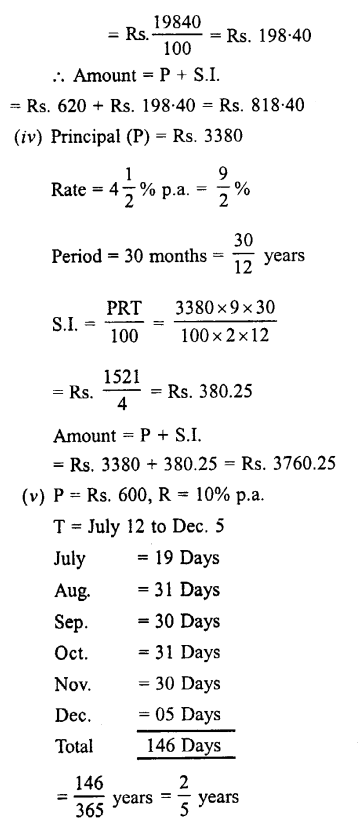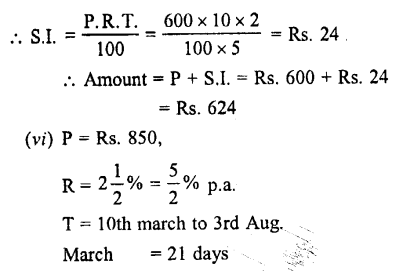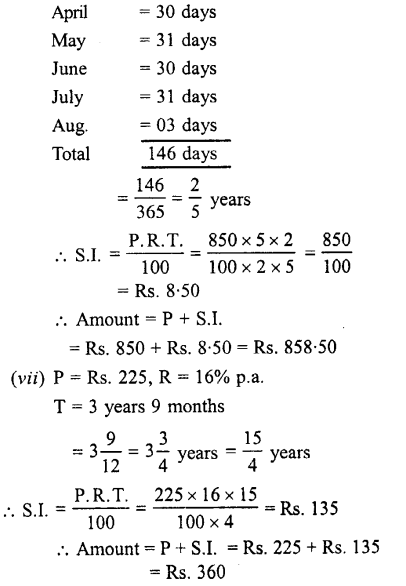Question 2.
On what sum of money does the S.I. for 10 years at 5% become Rs. 1,600 ?

Solution: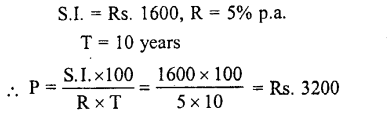Question 3.
Find the time in which Rs. 2,000 will amount to Rs. 2,330 at 11% p.a. ?

Solution:Question 4.
In what time will a sum of money double it self at 8% p.a ?

Solution: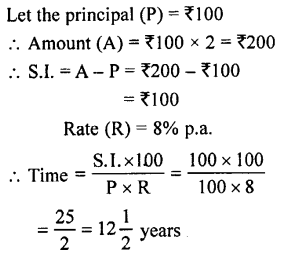Question 5.
In how many years will be ₹870 amount to ₹1,044, the rate of interest being 2$$\frac { 1 }{ 2 }$$ % p.a ?

Solution:Question 6.
Find the rate percent if the S.I. on ₹275 is 2 years is ₹22.

Solution: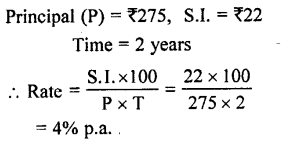Question 7.
Find the sum which will amount to ₹700 in 5 years at 8% rate p.a.

Solution: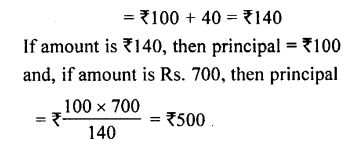Question 8.
What is the rate of interest, if ₹3,750 amounts to ₹4,650 in 4 years ?

Solution:Question 9.
In 4 years, ₹6,000 amount to ₹8,000. In what time will ₹525 amount to ₹700 at the same rate ?

Solution: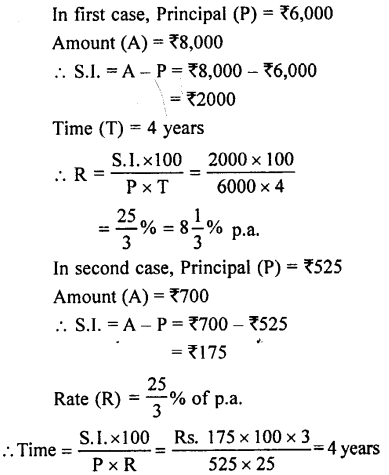Question 10.
The interest on a sum of money at the end of 2$$\frac { 1 }{ 2 }$$ years is $$\frac { 4 }{ 5 }$$ of the sum. What is the rate percent ?

Solution:Question 11.
What sum of money lent out at 5% for 3 years will produce the same interest as Rs. 900 lent out at 4% for 5 years ?

Solution: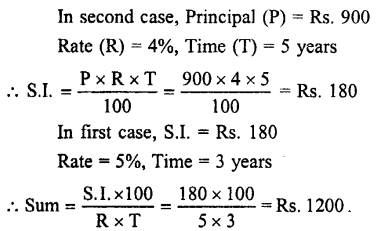Question 12.
A sum of Rs. 1,780 become Rs. 2,136 in 4 years,
Find :
(i) the rate of interest.
(ii) the sum that will become Rs. 810 in 7 years at the same rate of interest ?

Solution:Question 13.
A sum amounts to Rs. 2,652 in 6 years at 5% p.a. simple interest.
Find :
(i) the sum
(ii) the time in which the same sum will double itself at the same rate of interest.

Solution: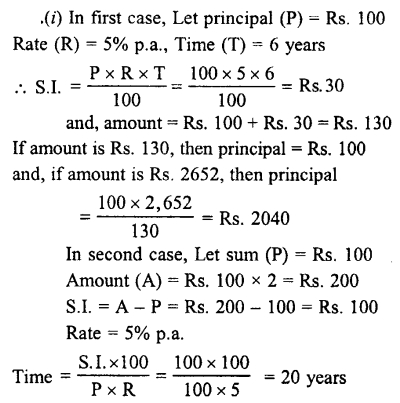Question 14.
P and Q invest Rs. 36,000 and Rs. 25,000 respectively at the same rate of interest per year. If at the end of 4 years, P gets Rs. 3,080 more interest than Q; find the rate of interest.

Solution:Question 15.
A sum of money is lent for 5 years at R% simple interest per annum. If the interest earned be one-fourth of the money lent, find the value of R.

Solution: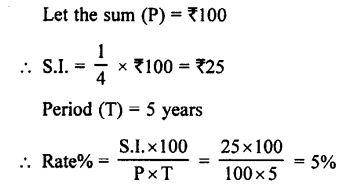Question 16.
The simple interest earned on a certain sum in 5 years is 30% of the sum. Find the rate of interest.

Solution: